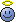# Finding the number of rational values a function can take

Gold Member

## Homework Statement

##f(x)## is a continuous and differentiable function. ##f(x)## takes values of the form ##^+_-\sqrt{I}## whenever x=a or b, (where ##I## denotes whole numbers) ; otherwise ##f(x)## takes real values. Also, ##|f(a)|\le |f(b)|## and ##f(c)=-1.5##. Graph of ##y=f(x)f'(x)##: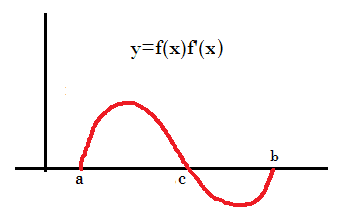The number of rational values that ##f(a)+f(b)+f(c)## can take is?

None

## The Attempt at a Solution

My teacher told me that the question has data insufficiency. But I don't know why.
##f(x)f'(x)=0## implies either ##f(x)## or ##f'(x)## or both are zero. At ##c## ##f'(x)## is zero since ##f(x)## is non zero. Hence, f(x) has a maxima or minima at ##x=c##.
Now, from ##a## to ##c##, either bothe the function and its derivative is positive or both are negative. Applying Rolle's theorem,
##{f'(k)}^2+f(k)f"(k)=0## since ##g(a)=(c)##.
But I don't seem to be getting anywhere.

Homework Helper
Looking at the plot, between a and c, f(x) and f'(x) are either both negative or both positive. Since f(c) = -1.5, we should assume both negative. Then from b to c, f(x) and f'(x) should have different signs, and we know that f(c) is negative, so assume f(x) <0 f'(x) >0.
So the range of f(a) is bounded, as is the range of f(b). And you know that they are integers.
f(c) is fixed, so it will not contribute to the number of possible solutions to f(a) + f(b) + f(c).
So, how many whole numbers can f(a) be? what about f(b)?

•Titan97
Gold Member
f(a) and f(b) is always irrational. (given in the question). So, for f(a) + f(b) + f(c) to be rational, f(a)=-f(b). Also, f(a)<0 and f(b)>0.

Homework Helper
f(a) and f(b) is always irrational. (given in the question). So, for f(a) + f(b) + f(c) to be rational, f(a)=-f(b). Also, f(a)<0 and f(b)>0.
The question says that f(a) and f(b) are roots of a whole number. So 1 and 0 are not out of the question.
if f(b) > 0, where does the function cross the axis, i.e. f(x)=0?

•Titan97
Staff Emeritus
Homework Helper
Gold Member
f(a) and f(b) is always irrational. (given in the question). So, for f(a) + f(b) + f(c) to be rational, f(a)=-f(b). Also, f(a)<0 and f(b)>0.
If ##\ I\ ## is a perfect square, then ##\ \sqrt{I}\ ## is rational.

•Titan97
Homework Helper
On interval (a,c), f(x) is either negative and decreasing or positive and increasing; which option makes it possible to continuously arrive at f(c) = -1.5?
On interval (c,b), f(x) is either negative and increasing or positive and decreasing; which option makes it possible to begin continuously from f(c) = -1.5?
Is it possible for there to be any value in (c,b) where f(x) = 0? What would the plot look like at that point?
What whole numbers have roots in your possible ranges?
What combinations of those values are possible after imposing ##|f(a)| \leq |f(b)|##?
I get a number less than 10.

Gold Member
f(c)<0. This means, f'(c)<0. This is only for x=c. How can you say its true for all ##x\in [a,c]##?

Staff Emeritus
Homework Helper
Gold Member
f(c)<0. This means, f'(c)<0. This is only for x=c. How can you say its true for all ##x\in [a,c]##?
The function is continuous, right.

If it changes sign to the left of c, then what value must it pass through?

Homework Helper
f(c)<0. This means, f'(c)<0. This is only for x=c. How can you say its true for all ##x\in [a,c]##?
You mean f'(c) = 0, as you said in your initial post.
Between a and c, neither f nor f' can be zero, as SammyS mentioned, so your possible values for f(a) are constrained.
Similarly, between c and b, neither f nor f' can be zero, so you are constrained for possible values at f(b).
Add in the additional constraints of |f(a)| ≤ |f(b)| and then counting only the rational sums does not leave many possible options.

•Titan97
Gold Member
I have been trying this problem for hours.
f'(c)=0, f(x)f'(x)>0 for ##x\in [a,c]##. But f(c)<0. So f(x) between a and c is also negative since function is continuous. But can't f(x) have a root between a and c?

Staff Emeritus
Homework Helper
Gold Member
I have been trying this problem for hours.
f'(c)=0, f(x)f'(x)>0 for ##x\in [a,c]##. But f(c)<0. So f(x) between a and c is also negative since function is continuous. But can't f(x) have a root between a and c?
What would that imply for ##\ y=f(x) f'(x) \ ## at that root ?

Gold Member
it implies that y=0

Staff Emeritus
Homework Helper
Gold Member
it implies that y=0
Does that shed some light on this problem?

Gold Member
I am confused. Here are some plots for f(x) which are possible: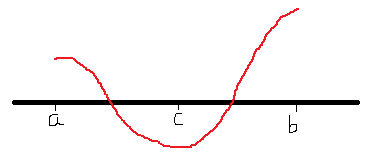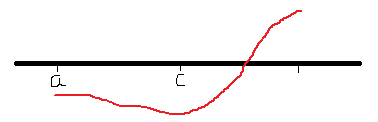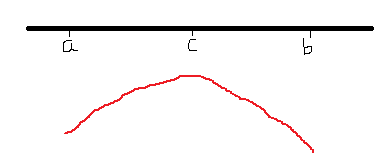Gold Member
Why can't there be a root? The graphs are for y=f(x) and not f(x)f'(x). There are no roots for y=f(x)f'(x) in a<x<c

Homework Helper
Gold Member
2022 Award
I am confused. Here are some plots for f(x) which are possible:
View attachment 86996

View attachment 86997

View attachment 86998
None of those look right to me. The curve given in the OP implies that in (a,c) f and f' have the same sign and in (c,b) they have the opposite sign.
But I do count three possibilities for the shape of f.

•Titan97
Gold Member
None of those look right to me. The curve given in the OP implies that in (a,c) f and f' have the same sign and in (c,b) they have the opposite sign.
But I do count three possibilities for the shape of f.
Ok. I will analyse the curve separately from a to c and from c to b.
From a to c:
From ##a## to ##c##, f(x) and f'(x) have same sign.
Since ##y=f(x)f'(x)## is not zero for any ##x\in (a,c)##, ##f(x)## and ##f'(x)##≠0. At ##x=a##, ##f(a)f'(a)=0##. So either ##f(a)## or ##f'(a)## or both are zero. giving 3 possibilities.
Since ##y>0## from ##a## to ##c##, and ##f(c)<0##, ##f'(x)## and ##f(x)## is forced to be negative. ##f(a)## may be zero.
So the curve can be either red or green: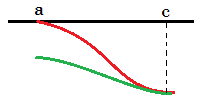Homework Helper
Gold Member
2022 Award
Ok. I will analyse the curve separately from a to c and from c to b.
From a to c:
From ##a## to ##c##, f(x) and f'(x) have same sign.
Since ##y=f(x)f'(x)## is not zero for any ##x\in (a,c)##, ##f(x)## and ##f'(x)##≠0. At ##x=a##, ##f(a)f'(a)=0##. So either ##f(a)## or ##f'(a)## or both are zero. giving 3 possibilities.
Since ##y>0## from ##a## to ##c##, and ##f(c)<0##, ##f'(x)## and ##f(x)## is forced to be negative. ##f(a)## may be zero.
So the curve can be either red or green
Yes, but you could make it clearer that the red curve isn't necessarily zero gradient at a. It need not have an inflexion between a and c.

•Titan97
Gold Member
ok. It could be zero. From c to b:
1. y=f(x)f'(x)<0 so f(x) and f'(x) have opposite signs
2. since f(c)<0, f'(x)>0 in (c,b) hence an increasing curve.
3. Again, y≠0 in (c,b) so the curve is entirely below/above x-axis.
4. f'(c)=0 and f(b)f'(b)=0.
So here are the possible curves: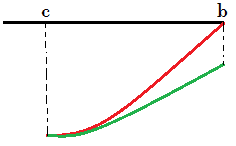Homework Helper
Gold Member
2022 Award
ok. It could be zero. From c to b:
1. y=f(x)f'(x)<0 so f(x) and f'(x) have opposite signs
2. since f(c)<0, f'(x)>0 in (c,b) hence an increasing curve.
3. Again, y≠0 in (c,b) so the curve is entirely below/above x-axis.
4. f'(c)=0 and f(b)f'(b)=0.
So here are the possible curves:
View attachment 87027
Right. But combining with (a,c), one of the four possibilities is ruled out in the OP, leaving 3.

•Titan97
Gold Member
Here are the possible curves: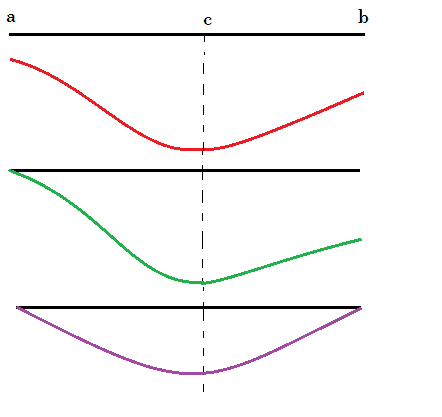Homework Helper
Gold Member
2022 Award
Here are the possible curves:

View attachment 87035
Right.
How about the original question? Not sure how much the diagrams help, but it's good to get them right.

•Titan97
Gold Member
i think f(a) and f(b) can only take values from 0 to -3/2.

Homework Helper
Gold Member
2022 Award
i think f(a) and f(b) can only take values from 0 to -3/2.
Yes. So combine that with the other info you have about f(a), f(b).

•Titan97
Gold Member
f(a) and f(b) can only take values of the form ##^+_-\sqrt{I}##. From 0 to -3/2, the values are 0,-√1,-√2.

Homework Helper
f(a) and f(b) can only take values of the form ##^+_-\sqrt{I}##. From 0 to -3/2, the values are 0,-√1,-√2.

•Titan97
Gold Member
f(a)=-√2 , f(b)=-√2
f(a)=-√1 , f(b)=-√1
f(a)=0 , f(b)=0
f(a)=0 , f(b)=-√1
f(a)=-√1 , f(b)=-√2
f(a)=0 , f(b)=-√2

Homework Helper
Gold Member
2022 Award
f(a)=-√2 , f(b)=-√2
f(a)=-√1 , f(b)=-√1
f(a)=0 , f(b)=0
f(a)=0 , f(b)=-√1
f(a)=-√1 , f(b)=-√2
f(a)=0 , f(b)=-√2
You listed six combinations, and I don't see any two that have the same sum.

Gold Member
what is that supposed to mean?

Homework Helper
Gold Member
2022 Award
what is that supposed to mean?
The question in the OP is
The number of rational values that f(a)+f(b)+f(c) can take is?
Given what you have found, why do you the answer is 3 instead of 6?

Gold Member
0 - 3/2 - √2 is not a rational number

•That's ok